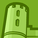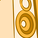QlikView App Dev

Discussion Board for collaboration related to QlikView App Development.

Announcements
QLIKWORLD LIVE! MAY 16 - 19TH, EARLY BIRD DISCOUNTS! REGISTER TODAY
cancel
Showing results for
Did you mean:Contributor III

Number of rows in straight table used in calculation condition

Hello,

I am writing with a question on how we can get the total number of rows in straight table and use that as part of calculation condition? Basically, I want to be able to limit the straight table to only show if there are 50K or less rows in that straight table not on my result set after filters (elaborated on Dynamic table sub-point)

I have 2 tables where I want to implement this:

1. Static table - dimensions and expressions are static. This has 8 dimensions.
2. Dynamic table - user has the ability to choose what dimensions or expressions they want to display, can go up from 1 to 32 dimensions.
1. Using just the number of rows returned by filters will not work in this situation in the case where a user selects dimensions and only returns 2 values, I want to still show that even if the dataset rows is still >50000 after any filters applied.

Things I've tried:

1. NoOfRows(TOTAL) - this doesn't seem to return the total number of rows when used outside of the expressions tab of that table so doing NoOfRows(TOTAL) <=50000 on the calculation condition doesn't work.
2. RowNo() - or other variations of this function also doesn't work for my use-case, pretty much same as NoOfRows
1. Also tried using it in the script to put a row number for my records, doesn't really work for me because of the scenario mentions in the Dynamic table sub-point.
3. Count(Distinct dimension1 & ... & dimensionN) - this one works for the static table although I notice significant slow down with 8 dimensions being processed.
1. I tried this same logic for the dynamic table but QlikView crashes due to lack of memory, I imagine because of the number of fields I try to concatenate (32).
1. Syntax: count(DISTINCT \$(=SubField(concat(distinct Dimension,  '|',  DimensionID), '|', 1)) & ... & \$(=SubField(concat(distinct Dimension,  '|',  DimensionID), '|', 32)))
1. Where \$(=SubField(concat(distinct Dimension,  '|',  DimensionID), '|', 1))  is my calculated dimension used on the straight table.

Thank you so much in advance,

Roebrich Perez

1 Solution

Accepted SolutionsContributor

Assuming the Object ID of your dynamic table is 'Custom Table':

1) Create a variable to hold the row count of your table (ie. vCustomTableRows)

2) Create a macro to update the variable.

Sub Update_vCustomTableRows

set TargetVariable = ActiveDocument.Variables("vCustomTableRows")

set TableBox = ActiveDocument.GetSheetObject( "Custom Table" )

CustomTableRows = TableBox.GetRowCount

TargetVariable.SetContent CustomTableRows , true

End Sub

3) In the Calculation Condition of your custom table (General properties tab), add the criteria:

vCustomTableRows <= 50000

4) Add an action to execute the macro, to any events that would affect the number of rows in your table.

a) Selection of dimensions in the dynamic table

b) Data filters

c) ???Contributor

Assuming the Object ID of your dynamic table is 'Custom Table':

1) Create a variable to hold the row count of your table (ie. vCustomTableRows)

2) Create a macro to update the variable.

Sub Update_vCustomTableRows

set TargetVariable = ActiveDocument.Variables("vCustomTableRows")

set TableBox = ActiveDocument.GetSheetObject( "Custom Table" )

CustomTableRows = TableBox.GetRowCount

TargetVariable.SetContent CustomTableRows , true

End Sub

3) In the Calculation Condition of your custom table (General properties tab), add the criteria:

vCustomTableRows <= 50000

4) Add an action to execute the macro, to any events that would affect the number of rows in your table.

a) Selection of dimensions in the dynamic table

b) Data filters

c) ???Community Browser